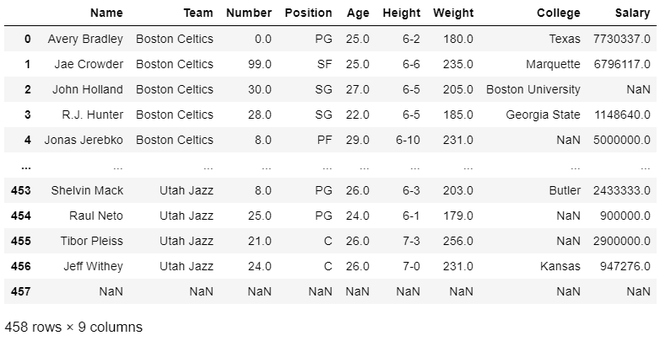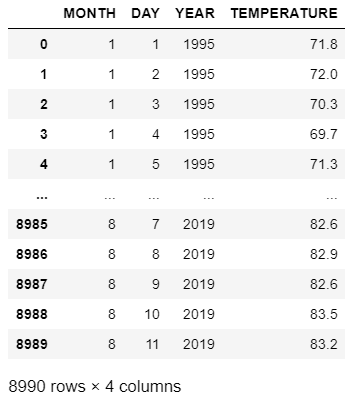Open in App
Not now

# Convert CSV to Pandas Dataframe

• Difficulty Level : Medium
• Last Updated : 02 Dec, 2020

In this article, we will discuss how to convert CSV to Pandas Dataframe, this operation can be performed using pandas.read_csv reads a comma-separated values (csv) file into DataFrame.

Example 1: In the below program we are going to convert nba.csv into a data frame and then display it.

## Python

 `# import pandas module ``import` `pandas as pd ``   ` `# making dataframe ``df ``=` `pd.read_csv(``"nba.csv"``) ``  ` `# output the dataframe``print``(df)`

Output:Example 2: Here is another example to convert a CSV dataset into pandas data frame.

## Python

 `# import pandas module ``import` `pandas as pd ``   ` `# making dataframe ``df ``=` `pd.read_csv(``"nba.csv"``) ``  ` `# output the dataframe``print``(df)`

Output:My Personal Notes arrow_drop_up